##### How to Subtract Minutes From a Time in PostgreSQL

Postgres offers numerous temporal data types that assist us in storing or manipulating the DateTime values efficiently. The built-in functions and operators of Postgres allow us to perform numerous tasks on DateTime values. Minus “-” is one of the built-in Postgres operators that allow us to subtract the specific minutes from a DateTime value.

The minus “-” operator has extended functionalities, however, this write-up explains how to subtract minutes from a time, date, timestamp, or interval using the “-” operator.

How to Subtract Minutes From a Time Using “-” Operator?

To subtract minutes from a time, specify the “-” sign between the given times. We can specify the intervals while subtracting the DateTime values.

Example 1: Subtracting Minutes From a Specific Time

In the below coding example, “19” minutes are subtracted from the given time:

`SELECT TIME '03:08' - INTERVAL '19 Minutes';`

In the above snippet, the TIME and INTERVAL represent built-in temporal data types.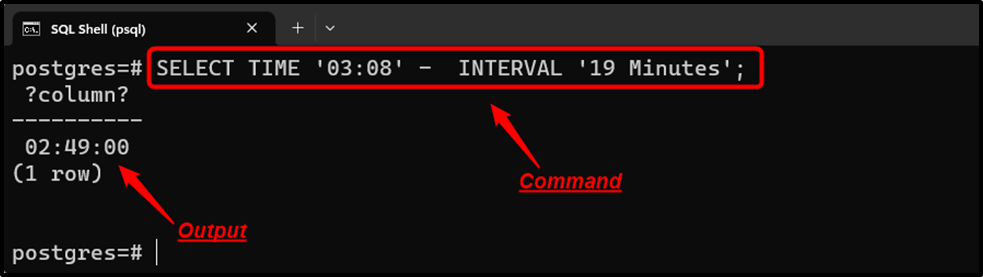Nineteen minutes have been subtracted from the given time.

Example 2: Subtracting Minutes From Current Time

In the following example code, “15” minutes are subtracted from the current time:

```SELECT CURRENT_TIME,
CURRENT_TIME - INTERVAL '15 Minutes' AS subtracted_time;```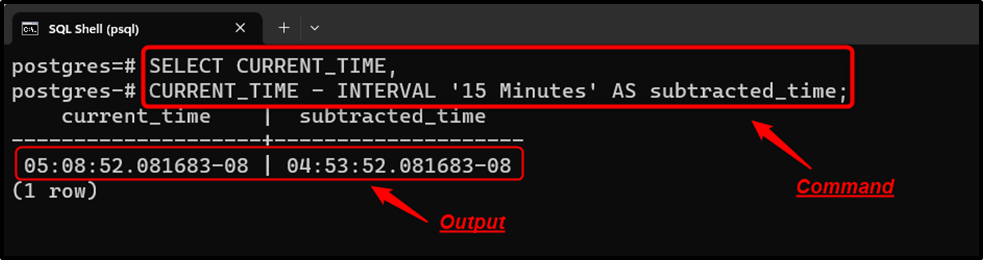The specified minutes have been subtracted from the current time.

Example 3: Subtracting Minutes From a Specific Timestamp

Let’s learn how to subtract “55” minutes from a particular timestamp:

`SELECT TIMESTAMP '2022-12-31 23:30:30' - INTERVAL '55 Minutes';`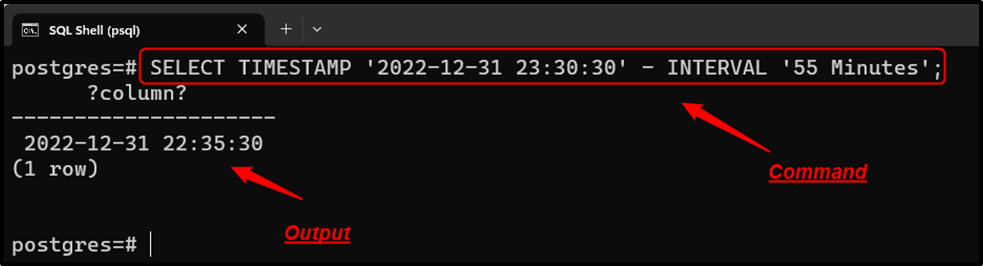The specified minutes have been successfully subtracted from the given timestamp.

Example 4: Subtracting Minutes From Current Timestamp

In this example, “15” minutes are subtracted from the current timestamp:

`SELECT CURRENT_TIMESTAMP - INTERVAL '15 Minutes';`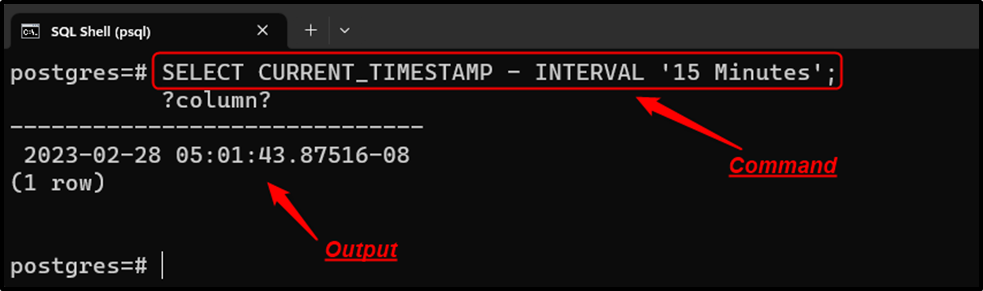Fifteen minutes have been successfully subtracted from the current timestamp.

Example 5: Subtracting Minutes From a Specific Interval

Let’s learn how to subtract minutes from a specific interval using the “-” operator:

`SELECT INTERVAL '5 years 4 days 2 hours 10 minutes' - INTERVAL '17 Minutes';`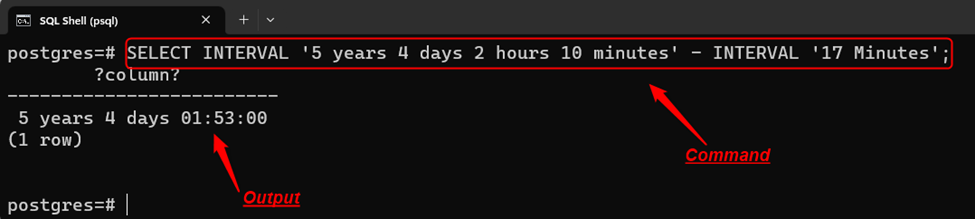“17” minutes have been successfully subtracted from the given interval using the “-” operator.

Example 6: Subtracting Minutes FROM Current Date

In the below snippet, “75” minutes are subtracted from the current date using the “-” operator:

`SELECT CURRENT_DATE - INTERVAL '75 Minutes';`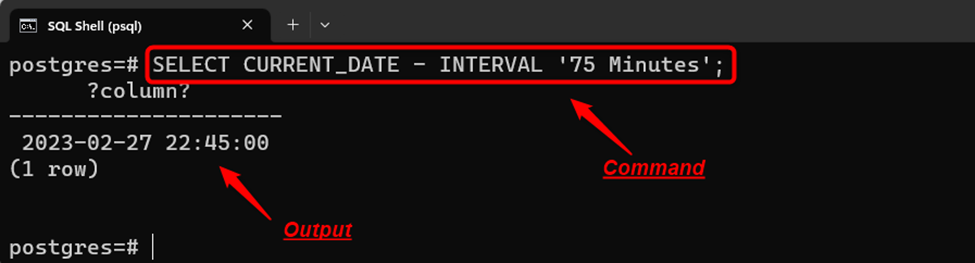The output demonstrates that the specified minutes have been successfully subtracted from the current date.

Example 7: Subtracting Seconds From Specific Minutes

In the below code, “130” seconds are subtracted from the given minutes using the “-” operator:

`SELECT TIME '00:09:00' - INTERVAL '380 Seconds';`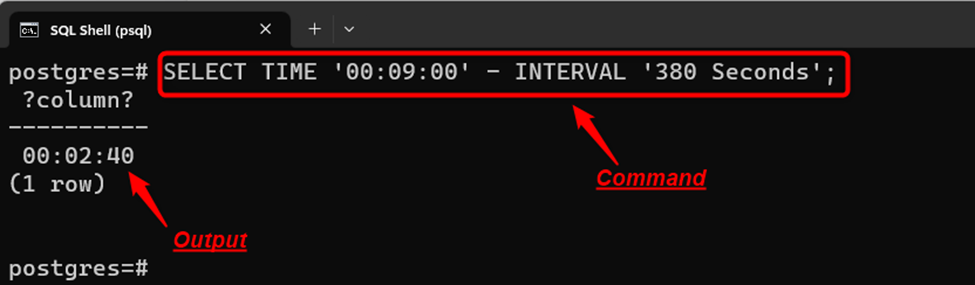“380” seconds have been subtracted from the given minutes.

Conclusion

In PostgreSQL, the “-” operator is used to subtract minutes from the current or specific DateTime values. Where the DateTime value can be a date, interval, time, or timestamp. We can specify the intervals while subtracting the DateTime values. The minus “-” operator has extended functionalities, however, in this post, we have explained a few use cases of the “-” operator, such as how to subtract minutes from a time, date, timestamp, or interval using the “-” operator.